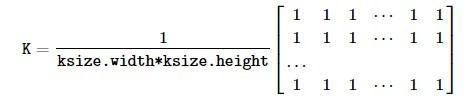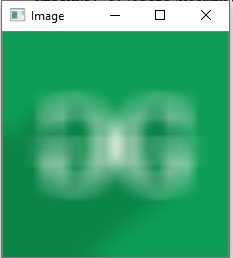# Python OpenCV | cv2.blur() method

• Difficulty Level : Easy
• Last Updated : 29 Nov, 2019

OpenCV-Python is a library of Python bindings designed to solve computer vision problems. `cv2.blur()` method is used to blur an image using the normalized box filter. The function smooths an image using the kernel which is represented as:Syntax: cv2.blur(src, ksize[, dst[, anchor[, borderType]]])
Parameters:
src: It is the image whose is to be blurred.
ksize: A tuple representing the blurring kernel size.
dst: It is the output image of the same size and type as src.
anchor: It is a variable of type integer representing anchor point and it’s default value Point is (-1, -1) which means that the anchor is at the kernel center.
borderType: It depicts what kind of border to be added. It is defined by flags like cv2.BORDER_CONSTANT, cv2.BORDER_REFLECT, etc
Return Value: It returns an image.

Image used for all the below examples:Example #1:

 `# Python program to explain cv2.blur() method  ` ` `  `# importing cv2  ` `import` `cv2  ` ` `  `# path  ` `path ``=` `r``'C:\Users\Rajnish\Desktop\geeksforgeeks\geeks.png'` ` `  `# Reading an image in default mode  ` `image ``=` `cv2.imread(path)  ` ` `  `# Window name in which image is displayed  ` `window_name ``=` `'Image'` ` `  `# ksize ` `ksize ``=` `(``10``, ``10``) ` ` `  `# Using cv2.blur() method  ` `image ``=` `cv2.blur(image, ksize)  ` ` `  `# Displaying the image  ` `cv2.imshow(window_name, image)  `

Output:Example #2:

 `# Python program to explain cv2.blur() method  ` ` `  `# importing cv2  ` `import` `cv2  ` ` `  `# path  ` `path ``=` `r``'C:\Users\Rajnish\Desktop\geeksforgeeks\geeks.png'` ` `  `# Reading an image in default mode  ` `image ``=` `cv2.imread(path)  ` ` `  `# Window name in which image is displayed  ` `window_name ``=` `'Image'` ` `  `# ksize ` `ksize ``=` `(``30``, ``30``) ` ` `  `# Using cv2.blur() method  ` `image ``=` `cv2.blur(image, ksize, cv2.BORDER_DEFAULT)  ` ` `  `# Displaying the image  ` `cv2.imshow(window_name, image)  `

Output:My Personal Notes arrow_drop_up
Recommended Articles
Page :